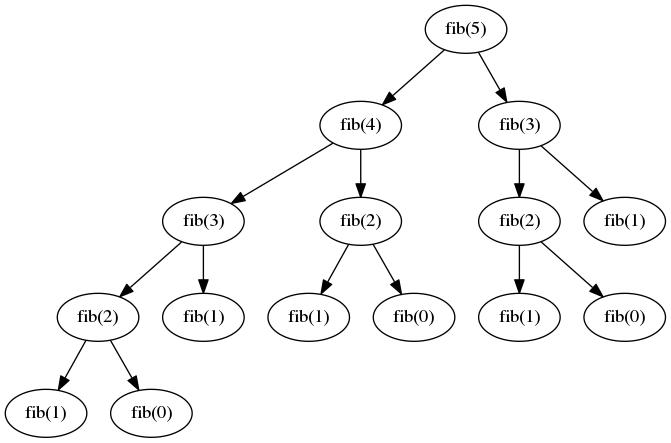# Digging in the depth of Fibonacci

Back

Not so long ago, I read an article on fibonacci. I suggested a more elegant solution to my eyes, but the problem kept bogging me. Let’s get into it!

## The naïve recursive approach

The first implementation, which reads like the mathematical formula, looks like this:

``````def fib(0), do: 1
def fib(1), do: 1
def fib(n), do: fib(n-1) + fib(n-2)``````

The main issue with this implementation is that the bigger the index you calculate, the exponentially bigger is number of operation needed. To show how, let’s look at the following graph of calls of the `fib(5)`:Fig 1: Recursive Fibonacci implementation function call graph

As you can see `fib(2)` is calculated 3 times, which is a inefficient. The number of recursive calls of `fib` will grow exponentially as we advance in the sequence. My computer start having big problems when attempting to calculate `fib(35)`. You could even get the result before you computer, which is a pretty bad sign! Let’s find another way to remove most of that calculation.

## Enters memoization

Memoization is an optimisation technique that speeds up algorithm by saving intermediate function calls which are used multiple times. In our case, if we calculate `fib(n)`, we’ll save every `fib(i)` with `i &lt; n`.

Elixir is not designed to tackle this kind of optimisation, but you can find a javascript implementation here

Although this technique will yield much better results at a benchmark, it is not yet an optimized version of the algorithm.

## Dynamic programming

The last algorithm optimization available is called dynamic programming. It seems like a complex word, and it covers a lot of techniques but in the case of Fibonacci, the algorithm is elegant and intuitive.

The first thing you have to ask yourself is: “How would I calculate `fib(5)`?” Let’s try to make it step by step:

``````fib(2) = fib(1) + fib(0) = 1 + 1 = 2
fib(3) = fib(2) + fib(1) = 2 + 1 = 3
fib(4) = fib(3) + fib(2) = 3 + 2 = 5
fib(5) = fib(4) + fib(3) = 5 + 3 = 8``````

For each step you only used 3 explicit variables, `i`, `fib(i)`, `fib(i - 1)`, `fib(i - 2)` and an implicite one, the sequence number we calculate `n` (5 in this case). It’s pretty simple to implement a function which will replicate this behavior:

``````def fib(0), do: 1
def fib(1), do: 1
def fib(n), do: dyna_fib(1, 1, n)

def fib_step(result, _, 1), do: result
def fib_step(fib_i_minus_one, fib_i_minus_two, i) do
fib_step(fib_i_minus_one + fib_i_minus_two, last, i - 1)
end``````

It’s a very fast implementation, it’s complexity drops to `O(n)`. This version calculates very easily the millionth element of the Fibonacci sequence!

## Using Maths at our advantage

As you might know, the Fibonacci sequence is a mathematical object. Maybe maths have some interesting formulas, you can find the most interesting formula in the end of this chapter

Fib(2n - 1) = F(n)² + F(n - 1)²

Fib(2n) = (2 F(n - 1) + F(n)) F(n)

Those formulas are a huge improvement! They would allow to jump indices of the sequence by a factor of 2! This will allow to reduce the complexity to `O(log(n))`, which is quite impressive. The implementation is straight forward:

``````def log_fib(0), do: 0
def log_fib(1), do: 1
def log_fib(2), do: 1
def log_fib(n) when rem(n, 2) == 1 do
# Fib(2n - 1) = F(n)² + F(n - 1)²
i = div((n + 1), 2)
first = log_fib(i)
second = log_fib(i - 1)
first * first + second * second
end
def log_fib(n) when rem(n, 2) == 0 do
# Fib(2n) = (2 * F(n - 1) + F(n)) * F(n)
i = div(n, 2)
first = log_fib(i)
(2 * log_fib(i - 1) + first) * first
end``````

## Using a library

Instead of getting all the problems with implementing an optimized version, you should use a library that does the job for you. Gnu MP is, as far as I know, the most optimized version of Fibonacci and uses a memoized version of the `O(log(n))` recursive algorithm.

I didn’t think I would get so deep when starting the article. I wanted to show off with dynamic programming, but in this case it’s not even the optimized version. The lesson of this article should be: always doubt on an opinion you have on a subject you don’t know well. We tend to take our opinions for truth, but if we confront them to a methodological and rigorous research process, they always prove to be, at best, misleading.

Discuss on twitter